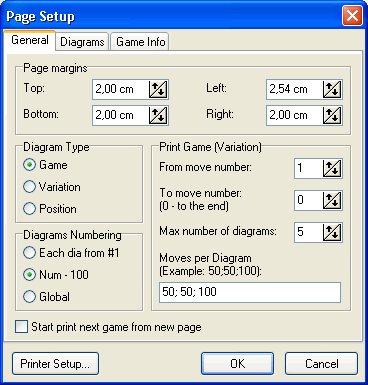# File: Page Setup: General'Page margins'. This group of parameters sets all four margins (in centimetres) for printed pages.

'Diagram type'. Defines which kind of printout you will have.

1. Game for print game, set of games or variation

2. Variation for print variations tree

'Diagram numbering'. It valid for game and variation diagram types. The parameter determines type of numbering for multidiagrams games.

• 'Each dia from #1' means that each diagram will restart numbering from the 1st move

• 'Num - 100'. The numbering continues from diagram to diagram, but there will print only two digits (without hundreds).

• 'Global'. Continuous numbering.

'Print Game (Variation)'.

'From move number'. It sets from which move the numbering will start. Default value is 1 (usual for the games printing). If you want to print something like variation from given position, change this parameter to the starting move number of the variation.

'To move number'. This parameter sets last move for printout. '0' means end of the game. Do not leave 0 for the print variations and subtrees of variations.

'Max number of Diagrams' Number of diagrams from which consists printout for the game. There is possible to set 'Moves per Diagram' option such as number of diagrams will be less than entered.

'Moves per Diagram' Determines number of moves per diagram. If blank, then number of moves for the game automatically divides by number of diagrams.

Examples:
'50;50;100' means 50 moves for diagrams 1 and 2 and 100 moves for other diagrams.
'5' means 5 moves for all diagrams, except last and all rest moves for last diagram.
More examples.

'Start print next game from new page' Useful only for print set of games.